Include Top

# CUSUM ARL

1. Click SigmaXL > Templates & Calculators > Control Chart Templates > Average Run Length (ARL) Calculators >CUSUM ARL. This template is also located at SigmaXL > Control Charts > Control Chart Templates> Average Run Length (ARL) Calculators >CUSUM ARL.

2. The default template settings are Specify = k & h, k parameter = 0.5, h parameter = 5, Fast Initial Response: FIR = 0, Method = Markov Chain.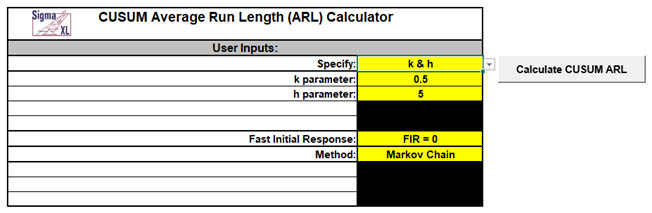Notes: Parameters to be specified will be shown in yellow highlight, otherwise they are hidden. The CUSUM parameter k is the reference (or slack) value, typically set to 0.5. It sets the size of mean shift (2k sigma) that you would like to detect quickly, so 0.5 denotes rapid detection of a shift in mean = 1 sigma. Alternatively, Woodall &Faltin  recommend larger k values (e.g., k = 0.9) to avoid false alarms and detect shifts of practical significance.

The CUSUM parameter h is the decision interval, typically set to 4 or 5.

FIR sets the initial CUSUM statistic so that it improves the sensitivity to a mean shift at startup. Note that if the process is in control when the CUSUM is started but shifts out of control later, the more appropriate ARL for such a case would be FIR=0. See Montgomery , pages 426-427.

Markov Chain approximation is fast and accurate to compute ARLs. Monte Carlo simulation allows you to assess robustness to nonnormality and also produces the table of Run Length Standard Deviation and Percentiles (scroll right to view).

For further details on the Markov Chain approximation see Hawkins  and Lucas . Monte Carlo simulation uses the Pearson Family of distributions to match the specified skewness and kurtosis.

The CUSUM ARL is for a two-sided chart with zero-state, i.e., the shift is assumed to occur at the start. The mean and standard deviation are also assumed to be known. This will not likely be the case in use, but is still useful for determining parameter settings and comparison of ARL across chart types.

All ARL calculations for CUSUM use a standardized in-control mean=0 and sigma=1.

3. Click the Calculate CUSUM ARL button to reproduce the ARL table and chart.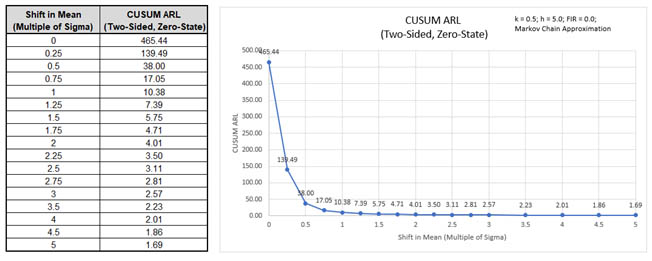The ARL0 (in-control ARL with 0 shift in mean) for the CUSUM chart with these settings is 465.44. The ARL1 for a small 1 sigma shift in mean is 10.38.

4. Now we will evaluate CUSUM with the same parameters, but use the Fast Initial Response option. Select Specify = k & h. Enter k parameter = 0.5, h parameter = 5, Fast Initial Response FIR = h/2, Method = Markov Chain.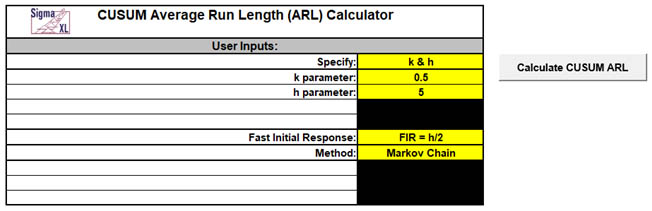5. Click the Calculate CUSUM ARL button to produce the ARL table and chart for these settings.The ARL0 for the CUSUM chart with these settings is 430.39 which is slightly lower than the FIR = 0 setting, so slightly higher false alarm rate. The ARL1 for a small 1 sigma shift in mean is 6.35 which is faster than the 10.38 for FIR = 0.

6. Now we will specify the CUSUM k parameter = 0.5 with a Shewhart ARL0 value of 370.4 and solve for the h parameter. Enter Specify = k & ARL0, k parameter = 0.5, In-Control Average Run Length (ARL0) = 370.4, Fast Initial Response FIR = 0, Method = Markov Chain.7. Click the Calculate CUSUM ARL button to produce the CUSUM Parameters, ARL table and chart for these settings.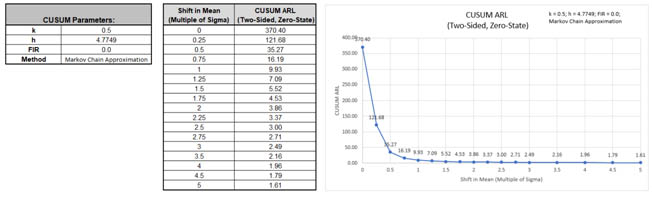The ARL0 for the CUSUM chart with these settings is 370.4 as specified. The h parameter solved to obtain this ARL0 value is 4.7749. The ARL1 for a small 1 sigma shift in mean is 9.93 so is much faster to detect than the ARL1 of 43.89 for Shewhart Individuals and close to the Monte Carlo ARL1 of 9.7 for Shewhart Individuals with 8 tests for special causes.

8. Next, we will specify a Shewhart ARL0 value of 370.4, with a desired optimization to detect a 1 sigma shift in mean and use Fast Initial Response. The calculator will solve for the optimal k and h parameters. Enter Specify = ARL0 & Shift, In-Control Average Run Length (ARL0) = 370.4, Shift in Mean to Detect (Multiple of Sigma) = 1, Fast Initial Response FIR = h/2, Method = Markov Chain.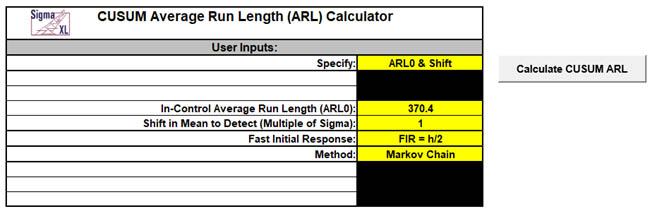Note: Since both k and h are solved, this takes about 20-30 seconds to compute.

9. Click the Calculate CUSUM ARL button to produce the CUSUM Parameters, ARL table and chart for these settings.The ARL0 for the CUSUM chart with these settings is 370.4 as specified. ARL1 for a small 1 sigma shift in mean is 6.08 so is much faster to detect than the ARL1 of 43.89 for Shewhart Individuals and faster than the Monte Carlo ARL1 of 9.7 for Shewhart Individuals with 8 tests for special causes. It is slightly faster to detect than the ARL1 of 6.76 for Time-Varying EWMA with Weight(Lambda) = .05. The solved parameters are k = 0.4111, h = 5.7051 and FIR = 2.8526.

10. As an alternative to using the CUSUM to rapidly detect small shifts in mean, Woodall & Faltin  recommend larger k values to avoid false alarms and detect shifts of practical significance. Enter Specify = k & h, k parameter = 0.9, h parameter = 4.65, Fast Initial Response FIR = 0, Method = Markov Chain.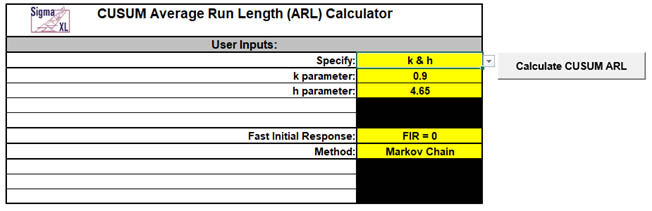11. Click the Calculate CUSUM ARL button to produce the CUSUM Parameters, ARL table and chart for these settings.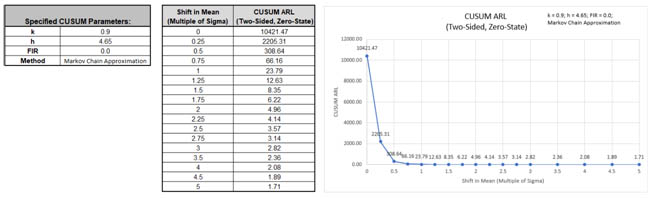This gives large ARL values for shift in mean <= 1 sigma and small ARLvalues for a shift in mean >= 1.5 sigma.

12. Now we will use Monte Carlo simulation to obtain approximate Run Length standard deviation and percentiles using the CUSUM k parameter = 0.5 with a Shewhart ARL0 value of 370.4. Enter Specify = k & ARL0, k parameter = 0.5, In-Control Average Run Length (ARL0) = 370.4, Fast Initial Response FIR = 0, Method = Monte Carlo, Number of Replications = 1e4, Skewness = 0, Kurtosis (Normal is 0) = 0.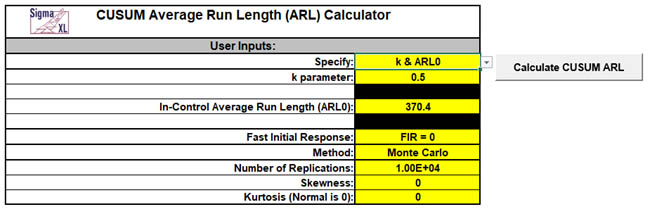Note: The CUSUM h parameter will be solved first using the Markov Chain approximation and assumes a Normal distribution, so will match the 4.7749 value previously calculated above (6).

13. Click the Calculate CUSUM ARL button to produce the CUSUM Parameters, Monte Carlo approximate ARL table, ARL chart and Run Length Standard Deviation and Percentiles table. Monte Carlo simulation with 10,000 (1e4) replications will take about a minute to run.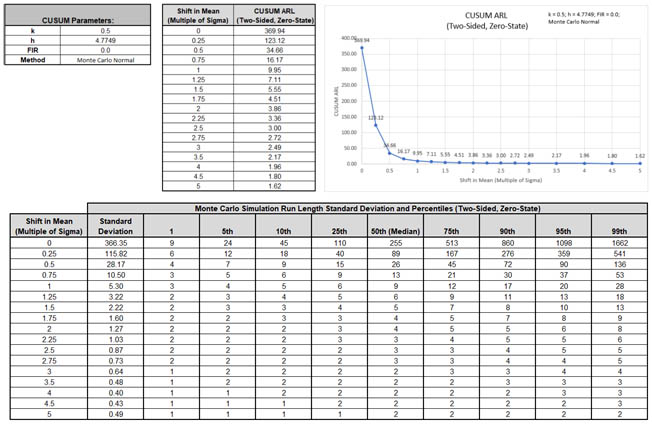The additional run length statistics show the large variation of run length values. The MRL0 = 255 (in-control median run length with 0 shift in process mean).

Note: The results will vary slightly since this is Monte Carlo simulation.

14. We will now assess robustness to nonnormality using Monte Carlo simulation and compare to Shewhart and EWMA charts. Enter Specify = k & ARL0, k parameter = 0.5, In-Control Average Run Length (ARL0) = 370.4, Fast Initial Response FIR = 0, Method = Monte Carlo, Number of Replications = 1e4, Skewness = 2, Kurtosis (Normal is 0) = 6.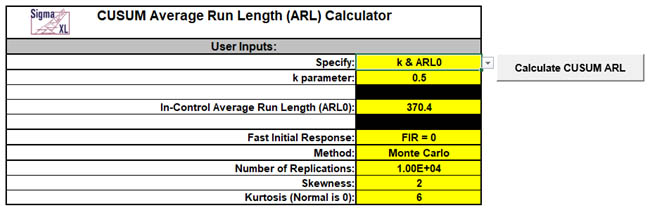15. Click the Calculate CUSUM ARL button to produce the CUSUM Parameters, Monte Carlo approximate ARL table, ARL chart and Run Length Standard Deviation and Percentiles table:ARL0 is approximately 161.4 which is a 2.3 x increase (370.4/161.4) in false alarms compared to Normal but is a much better performance than the ARL0 = 54.6 result for Shewhart Individuals.

It is, however, less robust to nonnormality than EWMA chart with Weight (Lambda) = 0.1 which had a Monte Carlo ARL0 = 266.4.

MRL0 is approximately 112.

Stoumbos and Reynolds  recommend setting h=6.148 (with k=0.5) as a way to improve the CUSUM robustness to non-normality.

# Template Notes:

1. Specify CUSUM parameters: k & h, k &ARL0 or ARL0& Shift using the drop-down list. Parameters to be specified will be shown in yellow highlight, otherwise they are hidden.
2. If applicable, enter the CUSUM parameter k. This is the reference (or slack) value, typically set to 0.5. It sets the size of mean shift (2k sigma) that you would like to detect quickly, so 0.5 denotes rapid detection of a shift in mean = 1 sigma. Alternatively, Woodall &Faltin  recommend larger k values (e.g., k = 0.9) to avoid false alarms and detect shifts of practical significance.
3. If applicable, enter the CUSUM parameter h. This is the decision interval, typically set to 4 or 5.
4. If applicable, enter the desired In-Control Average Run Length (ARL0). This will be the target ARL for mean shift = 0 and should be a large value to minimize false alarms, typically 370 to 500. The h parameter will be solved to achieve this ARL0, given a specified k value.
5. If applicable, enter the desired Shift in Mean to Detect (Multiple of Sigma). This will minimize ARL for the given shift. If FIR=0, then k will be shift/2. If FIR=h/2, then k will be optimized, requiring about 20-30 seconds to compute.
6. Select FIR=0 or FIR=h/2 using the drop-down list. This is the fast initial response (or headstart) value.
7. FIR sets the initial CUSUM statistic so that it improves the sensitivity to a mean shift at startup. Note that if the process is in control when the CUSUM is started but shifts out of control later, the more appropriate ARL for such a case would be FIR=0. See Montgomery , pages 426-427.
8. Select Method: Markov Chain or Monte Carlo using the drop-down list. Markov Chain approximation is fast and accurate to compute ARLs. Monte Carlo simulation allows you to assess robustness to nonnormality and also produces the table of Run Length Standard Deviation and Percentiles (scroll right to view).
9. For further details on the Markov Chain approximation see Hawkins  and Lucas . Monte Carlo simulation uses the Pearson Family of distributions to match the specified skewness and kurtosis.
10. If applicable, enter Number of Replications. 1000 (1e3) replications will be fast, approx. 10 seconds, but will have an ARL0 error approx. = +/- 10%; 10,000 (1e4) replications will take about a minute, with an ARL0 error = +/- 3.2%; 100,000 (1e5) replications will take about ten minutes, with an ARL0 error = +/- 1%.
11. If applicable, enter Skewness. Skewness must be >= 0. Skewness = 0 is symmetric.
12. If applicable, enter Kurtosis (Normal is 0). Also known as Excess Kurtosis, it must be >= Skewness^2 - 1.48. This is required to keep the distribution unimodal. If Skewness=0 and Kurtosis = 0, the distribution is normal.
13. Click the Calculate CUSUM ARL button to produce the ARL table and chart. If Monte Carlo was selected, the table of Run Length Standard Deviation and Percentiles will also be produced.
14. The CUSUM ARL is for a two-sided chart with zero-state, i.e., the shift is assumed to occur at the start. The mean and standard deviation are also assumed to be known. This will not likely be the case in use, but is still useful for determining parameter settings and comparison of ARL across chart types.
15. Due to the complexity of calculations, SigmaXL must be loaded and appear on the menu in order for this template to function. Do not add or delete rows or columns in this template.

# REFERENCES:

1. Hawkins, D. M. and Olwell, D. H. (1998), Cumulative Sum Charts and Charting for Quality Improvement (Information Science and Statistics), Springer, New York.
2. Lucas, J.M. and Crosier R.B. (1982), “Fast Initial Response for CUSUM Quality-Control Schemes: Give Your CUSUM A Headstart”, Technometrics 24, 199-205.
3. Montgomery, D.C. (2013), Introduction to Statistical Quality Control, Seventh Ed., Wiley.
4. Woodall, W. H. and Faltin, F.W. (2019), "Rethinking control chart design and evaluation", Quality Engineering 31, 596-605.
5. Stoumbos, Z. G. and Reynolds, M.R. Jr. (2004), “The Robustness and Performance of CUSUM Control Charts Based on the Double-Exponential and Normal Distributions”, In: Lenz, H. J., Wilrich, P. T. (eds) Frontiers in Statistical Quality Control 7, Physica, Heidelberg, 79-100.

# Web Demos

Our CTO and Co-Founder, John Noguera, regularly hosts free Web Demos featuring SigmaXL and DiscoverSim